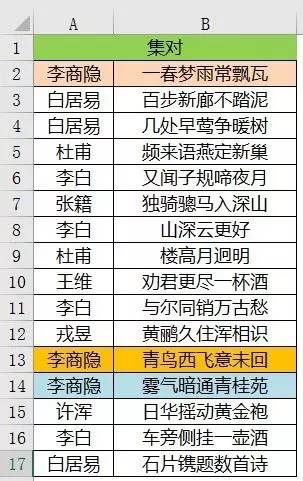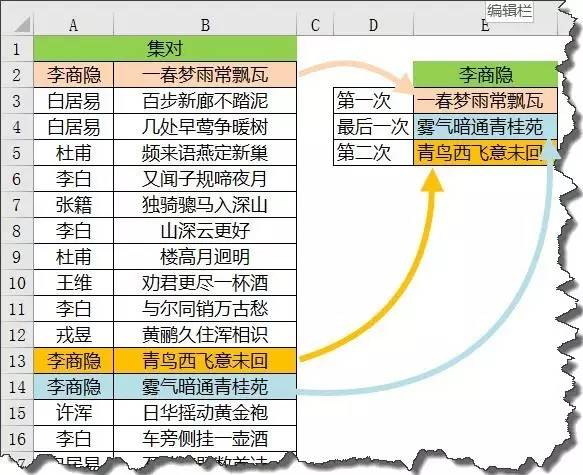# INDEX+SMAll+IF，经典查找三剑客

=INDEX(区域,SMALL(IF(条件,行号数组,4^8),ROW(A1)))1，李商隐第一次出现时的B列值；

2，李商隐最后一次出现时的B列值；

3，李商隐第2次出现时候的B列值。前面两个问题是比较常见的，我们简要一看。

=VLOOKUP(E2,A2:B17,2,FALSE)

=LOOKUP(1,0/(A2:A17=E2),B2:B17)

{=INDEX(B\$2:B\$17,SMALL(IF(A\$2:A\$17=E\$2,ROW(\$1:\$16),4^8),2))}

IF(A\$2:A\$17=E\$2,ROW(\$1:\$16),4^8)

{1;65536;……;65536;12;13;65536;65536;65536}